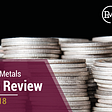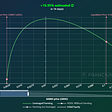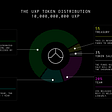This is a guide unpacking an institutional trader’s view of options trading. Thanks to Greg at Genesis Volatility!

# Options VS Delta-One

A big advantage and disadvantage of trading options is the vast number of instruments to choose from.

When trading underlying instruments (also known as “Delta-One”), the decision surface is rather simple. Will the instrument go up or down and is there a yield associated with holding the instrument?

Options provide non-linear payoff structures and are affected by multiple factors: time until expiration, chosen strike price, interest rates (risk-free and dividend), underlying asset prices, and implied volatility.

We will visit these factors in more detail later but for now just be aware that options have a multitude of variables which must be considered.

# What is implied volatility and why is it so important?

Implied volatility is the one factor that isn’t known upfront when pricing an option.

Options are traded in dollars and implied volatility can be determined (“backed out”) when dollar prices are combined with the other factors (strike price, time until expiration, underlying asset price, and interest rates can be readily observed in the market)

The last key input — implied volatility — is determined through an interactive and probabilistic process.

Traders can input an implied volatility “guess”, along with the other known parameters, into a formula — usually the Black-Scholes formula. Given an implied volatility, this formula will resolve into an option dollar price; traders will then iterate the implied volatility estimate until the Black-Scholes price output matches observed option-prices in the market.

We have now determined the option’s implied volatility — “implied” because the option price is implying a certain volatility input.

Implied volatility is usually quoted in terms of one annual standard deviation of continuously compounded returns — this is the Black-Scholes implied volatility.

Practitioners will commonly observe that normal price distributions and continuously compounded returns don’t always hold true, yet Black-Scholes implied volatility are still often quoted because the short-falls are simple and well understood.

Why use implied volatility?

Traders typically translate option dollar prices into implied volatility values in order to compare apples to apples.

An example will help us illustrate this point.

Assume the underlying asset price is \$3,708. What’s cheaper?

1. An option expiring in 175 days with a strike price of 25,000 trading for \$36.60 OR
2. An option expiring in 14 days with a strike price of 4,000 trading for \$154.30

Clearly \$154.30 is a higher price than \$36.60, but with so many other factors between the two options, how can we possibly compare the two prices without normalizing all the variables?

This is where implied volatility comes in. We are able to translate all the known variables — including the dollar cost of the options — into a standard value that is directly comparable.

The implied volatility of option 1 = 122% and the implied volatility of option 2 = 89%; therefore the option with the higher price tag (\$154.60) is actually cheaper than the (\$36.60) option in terms of implied volatility.

Why is lower implied volatility cheaper than higher implied volatility?

Expected value is the answer to this question.

Assets price returns, for the most part, are unpredictable — at least in theory — when pricing options. Options are also “one-way” bets from the buyer’s perspective, meaning that the most an option buyer can lose is the initial cash-outlay paid for the option (and any associated interest that would have been earned). Yet the buyer of a “ Call Option” — theoretically — has unlimited upside profit, since the underlying asset can theoretically rally infinitely higher.

Due to the nature of one-way bets, assets with higher volatility have a higher probability of seeing massive price changes. These price changes can make options extremely valuable.

EXAMPLE 1

Let’s see an example: (Ignoring interest rates)

1. Assume we are pricing a call with \$100 strike that expires tomorrow.
2. Assume the underlying asset has a 20% chance of being worth any 5 prices tomorrow: \$60, \$80, \$100, \$120, \$140
3. What value can the option be worth at expiration, respectively? \$0, \$0, \$0, \$20, \$40?
4. Given these outcomes, the price of the option today has an expected value (aka the probability weighted value)

[\$0 * .20] + [\$0 * .20] + [\$0 * .20] + [\$20 * .20] + [\$40 * .20] = \$12

EXAMPLE 2

Let’s try another example but this time with higher volatility:

1. Assume we are pricing a call with \$100 strike that expires tomorrow.
2. Assume the underlying asset has a 20% chance of being worth any 5 prices tomorrow: \$0, \$50, \$100, \$150, \$200
3. What value can the option be worth at expiration, respectively? \$0, \$0, \$0, \$50, \$100?
4. Given these outcomes, the price of the option today has an expected value (aka the probability weighted value)

[\$0 * .20] + [\$0 * .20] + [\$0 * .20] + [\$50 * .20] + [\$100 * .20] = \$30

Notice that increasing the distribution of returns increases the value of an option.

This is why implied volatility (aka the implied distribution) dictates the prices of options.

# The Volatility Surface

Why do two options, on the same underlying asset, have different implied volatilities? Shouldn’t one asset have one implied volatility?

This is a GREAT question and it brings us to the volatility surface.

Term structure and Skew represent the deviation of implied volatility when extending to different maturities (vertical), and different strikes (horizontal), respectively.

# Term Structure

Volatility has a very nice trading property associated with it, which is “mean reverting.” This means that in the long run, volatility will tend back towards the median.

This “mean reversion” becomes extremely pronounced in the term structure (different implied volatilities for different expirations).

In different environments we will see different term structures.

In a high volatility environment, volatility is high RIGHT NOW; but in the long-term, volatility will reflect the median regularly.

This high-volatility environment causes “Backwardation,” meaning short-term maturities will have higher implied volatility, and longer-term maturities will have lower implied volatility, because the market expects volatility mean reversion.

In low-volatility environments we will witness “Contango,” meaning short-term maturities will be priced with lower volatility and longer-term maturities will have higher implied volatility.

This is, once again, because the market expects (and therefore prices) mean reversion.

# Skew

Skew reflects implied volatility pricing deviations for options with different strikes, despite having the same maturities.

This phenomenon isn’t a result of mean reversion but of “Volatility Path”. This becomes very clear with diversified indexes. Put options will have more implied volatility as the strikes become farther “out-of-the-money”.

There are two underlying reasons for this:

First, if the market crashes, it tends to crash with more velocity than a market rise. The farther the crash, the bigger the panic — which leads to increased volatility and protective put demand.

The other factor is due to correlation: systematic risks (such as a global recession) affect all companies at once, therefore the protection offered from diversification diminishes and correlations become nearly one-to-one.

This lack of protection from diversification increases volatility.

Together, the affects become partially priced in, ahead of time, in the form of higher implied volatility for farther OTM options.

The reverse is true for equity index call options. Markets increase in stable times, stable times offer company diversification since companies all go their own ways. Stable times also reduces the probability of erratic price jumps.

Taken together, equity call options are priced with lower implied volatility the farther OTM they are.

These topics are a brief introduction to volatility trading and related concepts.

Something to keep in mind: Different assets have different volatility behaviors and react to different environments in their own ways.

Also, “Volatility Paths” are dynamic and therefore require constant assessment.

Traders can also trade “Relative Volatility.” For example, a trader can buy Ethereum call options and finance those by selling Bitcoin call options, expecting a change in correlation, volatility, relative strength, or all of the above.

We’ve also noticed an inverse relationship for crypto volatility, compared to equity index volatility: crypto EXPLODES higher and DRIFTS DOWN lower (over longer time periods). We therefore often see call options become more expensive than similar put options.

This phenomenon provides a sustainable income for “Covered Call” strategies that is not found in stocks. Check out Friktion’s piece on How Covered Calls Work to learn more.

Start earning @ app.friktion.fi/income

Still have questions? Find us in Discord!

--

--

--

## More from Friktion

Friktion brings high quality portfolio construction and dynamic risk management to DeFi.

Love podcasts or audiobooks? Learn on the go with our new app.

## GDP forecast Q4 2021: Strong rebound will be a brief respite## MAR 14. Another possible peace talk…## The Precious Metals Week in Review — July 27th, 2018## FEB 8. Crude oil price fell big with US/Iran talks## Africa’s Financial Future## The Radix Market View: Cautious Optimism## Friktion

Friktion brings high quality portfolio construction and dynamic risk management to DeFi.

## Pseudo-Delta Neutral Hedging Experiment 1## UXD Protocol Review## Friktion Auction Recap